##### Statistics For DummiesIn statistics, there are two important ideas regarding sample size and margin of error. First, sample size and margin of error have an inverse relationship. Second, after a point, increasing the sample size beyond what you already have gives you a diminished return, because the increased accuracy will be negligible.

The relationship between margin of error and sample size is simple: As the sample size increases, the margin of error decreases. This relationship is called an inverse because the two move in opposite directions. If you think about it, it makes sense that the more information you have, the more accurate your results are going to be (in other words, the smaller your margin of error will get). (That assumes, of course, that the data were collected and handled properly.)

## A simple example

Suppose that the Gallup Organization’s latest poll sampled 1,000 people from the United States, and the results show that 520 people (52%) think the president is doing a good job, compared to 48% who don’t think so. First, assume you want a 95% level of confidence, so you find z* using the following table.
Percentage Confidence z*-Value z*-Values for Selected (Percentage) Confidence Levels 80 1.28 90 1.645 95 1.96 98 2.33 99 2.58
From the table, you find that z* = 1.96.

The number of Americans in the sample who said they approve of the president was found to be 520. This means that the sample proportion,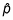is 520 / 1,000 = 0.52. (The sample size, n, was 1,000.) The margin of error for this polling question is calculated in the following way: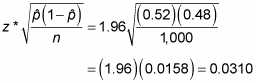According to this data, you conclude with 95% confidence that 52% of all Americans approve of the president, plus or minus 3.1%.

Using the same formula, you can look at how the margin of error changes dramatically for samples of different sizes. Suppose in the presidential approval poll that n was 500 instead of 1,000. Now the margin of error for 95% confidence is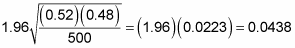which is equivalent to 4.38%. If n is increased to 1,500, the margin of error (with the same level of confidence) becomes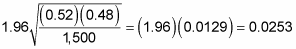or 2.53%. Finally, when n = 2,000, the margin of error is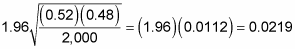or 2.19%.

## Balancing accuracy and cost

Looking at these different results, you can see that larger sample sizes decrease the margin of error, but after a certain point, you have a diminished return. Each time you survey one more person, the cost of your survey increases, and going from a sample size of, say, 1,500 to a sample size of 2,000 decreases your margin of error by only 0.34% (one third of one percent!) — from 0.0253 to 0.0219. The extra cost and trouble to get that small decrease in the margin of error may not be worthwhile. Bigger isn’t always that much better.